# OECD Trust in government data analysis 6 - Multiple linear regression using R - log(per capita GDP) and inflation rate and iso.Photo by Jim Cooke on Unsplash

This post is following of the above post.

So far previous posts, GDP nor per capita GDP cannot explain Trust in governmnet very well. I will add more variables.

I have CPI, inflation data like below.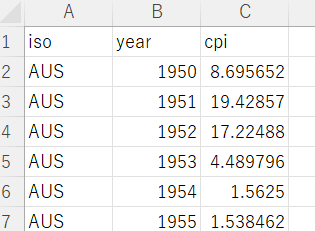I got this data from OECD web site. cpi means infration rate.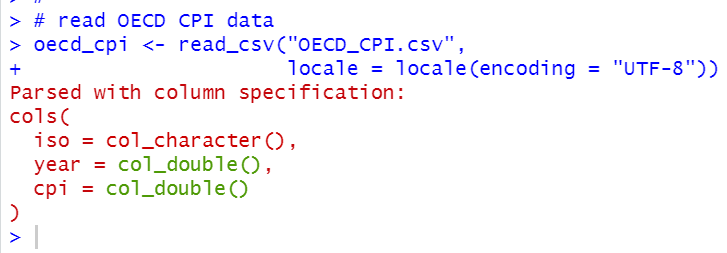I use inner_join() function to merge dataframe "df" and dataframe "oecd_cpi".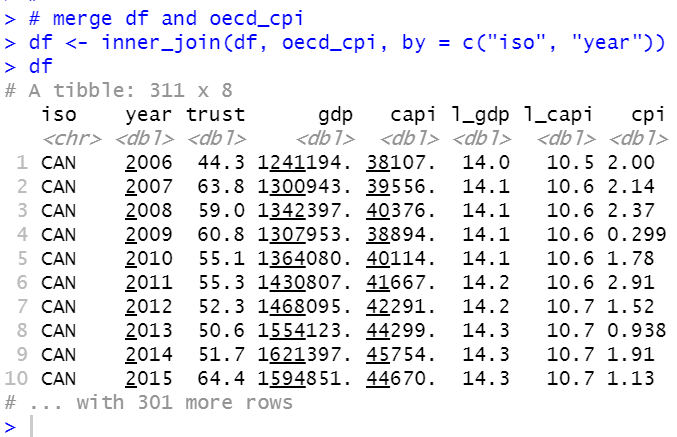Let's see correlation with trust, l_gdp, l_capi and cpi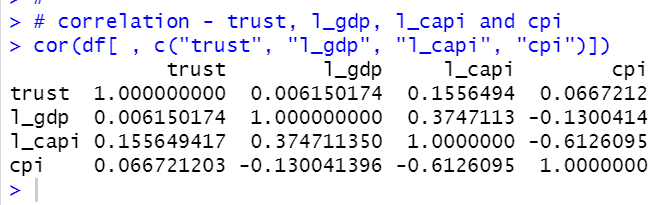l_capi and cpi has -0.61 correlation. and it is the absolutely largest correlation.

Let's see scatter plots matrix.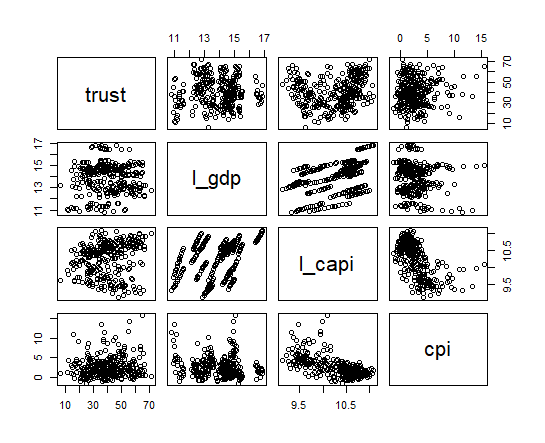Les's make a liear regression model. In this time, I select l_capi, cpi and iso for variables.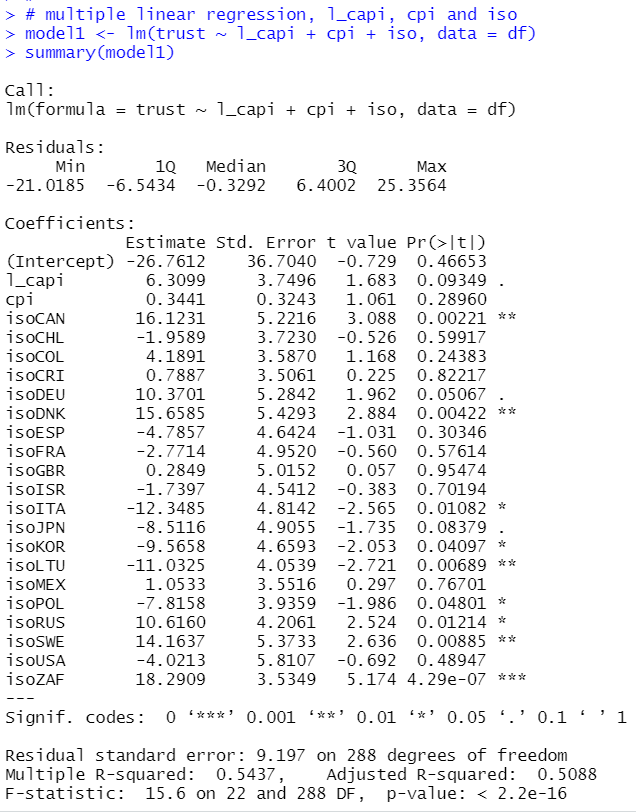I see l_capi's coefficient is 6.3099 with p-value 0.093. So, l_capi is statistically significant at 10% level. If cpi(infration rate) is fixed, more l_capi(log(per capita GDP)) makes more trust in government. It is natual.

I see some iso(country) has significant coefficients. Let's see it.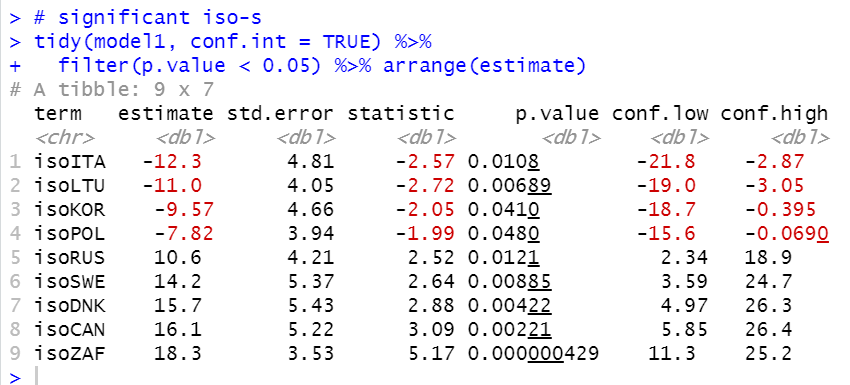ITA, LTU, KOR and POL have statistically low Trust in government if l_capi and cpi are fixed.

RUS, SWE, DNK, CAN and ZAF have statistically high Trus in government if l_capi and cpi are fixed.

That's it. Thank you!

Next post is